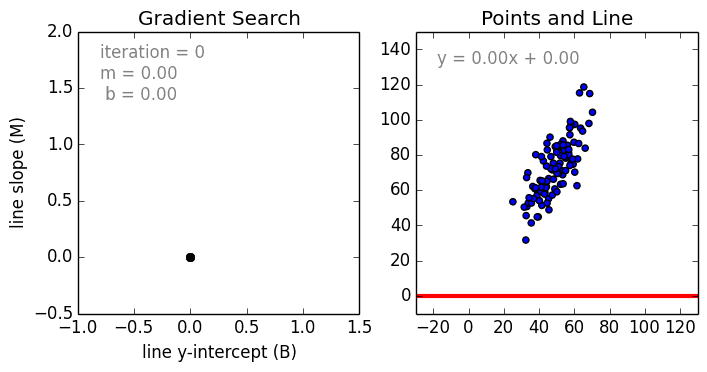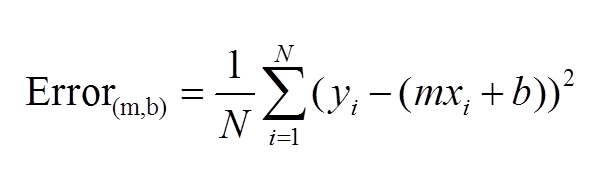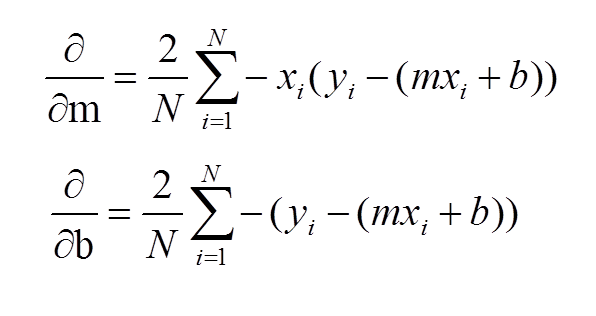# Linear Regression by using Gradient Descent Algorithm: Your first step towards Machine Learning.

So the hype is still on Machine Learning is everywhere. No matter if you’re from academia, software developer, advocate or from any background. You must have at least have some basic knowledge of machine learning in order to cope up with the most significant technology of mankind. Getting started with machine learning might be intimidating, but I have taken a small step to make you understand machine learning quite easy and in a simpler manner.

In order to ahead start with machine learning try to first learn about “Linear Regression” and code your own program from scratch using Python. Linear regression is very simple yet most effective supervised machine learning algorithm borrowed from statistics. This algorithm works on the underlying principle of finding an error. There are various “algebraic method” that can be used to solve linear regression problem, but here we are using Gradient Descent.

Why Gradient Descent?
Because it’s one of the best optimization methods that we can use to solve the various machine learning problem. eg. Logistic Regression, Neural Network. but here we are going to discuss about Linear Regression.

Here is some simplified definition of your understanding.

### What is Linear Regression?

#1 It’s a supervised machine learning algorithm which learns from given x dependent variable and Y as quantifiable variable and predicts New Y from given new X.
#2 It works in a way such that it finds the best fit line from the given data.
Clearly stated, the goal of linear regression is to fit a line to a set of points. Consider the following graph.https://raw.githubusercontent.com/mattnedrich/GradientDescentExample/master/gradient_descent_example.gif

To do this we’ll use the standard y = mx + b slope equation where m is the line’s slope and b is the line’s y-intercept. To find the best line for our data, we need to find the best set of slope m and y-intercept b values.

### What is Gradient Descent ?

Gradient descent is an optimization algorithm used to find the values of parameters (coefficients) of a function (f) that minimizes a cost function (cost).

To understand in an simpler way,let’s us take the example Suppose you are at the top of a mountain, and you have to reach a lake which is at the lowest point of the mountain. A twist is that you are blindfolded and you have zero visibility to see where you are headed. So, what approach will you take to reach the lake?

The best way is to check the ground near you and observe where the land tends to descend. This will give an idea in what direction you should take your first step. If you follow the descending path, it is very likely you would reach the lake.

At this point of time i hope you understood the fundamental concept of Linear Regression and Gradient Descent. Now let’s get into hands on with coding part.

A standard approach to solving this type of problem is to define an error function (also called a cost function) by incorporating gradient descent function we can achieve that measures how good fit a given line is.This function will take in an (m,b) pair and return an error value based on how well the line fits our data. To compute this error for a given line, we’ll iterate through each (x,y) point in our data set and sum the square distances between each point’s y value and the candidate line’s y value (computed at mx + b). It’s conventional to square this distance to ensure that it is positive and to make our error function differentiable.

### #! Remember this Sum of Square Error Equation.Equation of Sum of Square Error

To run gradient descent on this error function, we first need to compute its gradient. The gradient will act like a compass and always point us downhill. To compute it, we will need to differentiate our error function. Since our function is defined by two parameters (`m` and `b`), we will need to compute a partial derivative for each. These derivatives work out to be:

### #! Remember this Sum of Square Error Equation Partial Derivaties.Partial derivatives of Sum of Squared error.

The `learningRate` variable controls how large of a step we take downhill during each iteration. If we take too large of a step, we may step over the minimum. However, if we take small steps, it will require many iterations to arrive at the minimum.

Perhaps, that’s it you need to know the concept in order to understand Gradient Descent. Follow the github link, were I kept an example dataset for you, that show number of study hours effect in score in the subject or vice verse. This is how the whole code looks like. Click here to get the code to know more.

### Ending Note:

Example code for the problem described above can be found here

I chose to use linear regression example above for simplicity. We used gradient descent to iteratively estimate `m` and `b`, however we could have also solved for them directly. My intention was to illustrate how gradient descent can be used to iteratively estimate/tune parameters, as this is required for many different problems in machine learning.

### Credits:

1. An Introduction to Gradient Descent and Linear Regression
2. 2. Siraj Raval Youtube Channel